##### Trigonometry For DummiesThe first step to finding the trig function value of one of the angles that’s a multiple of 30 or 45 degrees is to find the reference angle in the unit circle. When the reference angle comes out to be 0, 30, 45, 60, or 90 degrees, you can use the function value of that angle and then figure out the sign of the angle in question. Use the following tables to find the reference angle.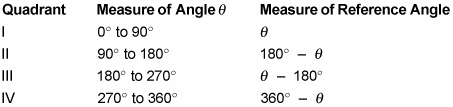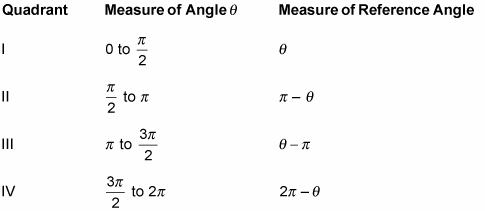All angles with a 30-degree reference angle have trig functions whose absolute values are the same as those of the 30-degree angle. The sines of 30, 150, 210, and 330 degrees, for example, are all either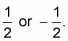The sine values for 30, 150, 210, and 330 degrees are, respectively,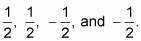All these multiples of 30 degrees have an absolute value of 1/2.

Likewise, using a 45-degree angle as a reference angle, the cosines of 45, 135, 225, and 315 degrees are all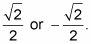In general, you can easily find function values of any angles, positive or negative, that are multiples of the basic (most common) angle measures.

Here’s how you assign the sign.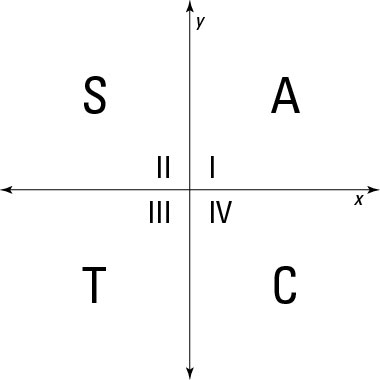Assigning positive and negative functions by quadrant.

The following rule and the above figure help you determine whether a trig-function value is positive or negative. First, note that each quadrant in the figure is labeled with a letter. The letters aren’t random; they stand for trig functions.

Reading around the quadrants, starting with QI and going counterclockwise, the rule goes like this: If the terminal side of the angle is in the quadrant with letter

```  A: All functions are positive
S: Sine and its reciprocal, cosecant, are positive
T: Tangent and its reciprocal, cotangent, are positive
C: Cosine and its reciprocal, secant, are positive```

In QII, only sine and cosecant are positive. All the other function values for angles in this quadrant are negative — and the rule continues in like fashion for the other quadrants.

A nice way to remember A-S-T-C is “All Students Take Calculus”.